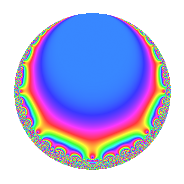Properties

 Label 128.4.a.eLevel 128 Weight 4 Character orbit 128.a Self dual Yes Analytic conductor 7.552 Analytic rank 1 Dimension 2 CM No Inner twists 1

Related objects

Newspace parameters

 Level: $$N$$ = $$128 = 2^{7}$$ Weight: $$k$$ = $$4$$ Character orbit: $$[\chi]$$ = 128.a (trivial)

Newform invariants

 Self dual: Yes Analytic conductor: $$7.55224448073$$ Analytic rank: $$1$$ Dimension: $$2$$ Coefficient field: $$\Q(\sqrt{3})$$ Coefficient ring: $$\Z[a_1, a_2, a_3]$$ Coefficient ring index: $$2^{2}$$ Fricke sign: $$-1$$ Sato-Tate group: $\mathrm{SU}(2)$

$q$-expansion

Coefficients of the $$q$$-expansion are expressed in terms of $$\beta = 4\sqrt{3}$$. We also show the integral $$q$$-expansion of the trace form.

 $$f(q)$$ $$=$$ $$q$$ $$+ ( -2 + \beta ) q^{3}$$ $$+ ( -2 - 2 \beta ) q^{5}$$ $$+ ( -4 - 2 \beta ) q^{7}$$ $$+ ( 25 - 4 \beta ) q^{9}$$ $$+O(q^{10})$$ $$q$$ $$+ ( -2 + \beta ) q^{3}$$ $$+ ( -2 - 2 \beta ) q^{5}$$ $$+ ( -4 - 2 \beta ) q^{7}$$ $$+ ( 25 - 4 \beta ) q^{9}$$ $$+ ( -46 - \beta ) q^{11}$$ $$+ ( -50 + 6 \beta ) q^{13}$$ $$+ ( -92 + 2 \beta ) q^{15}$$ $$+ ( 46 + 12 \beta ) q^{17}$$ $$+ ( -2 - 7 \beta ) q^{19}$$ $$-88 q^{21}$$ $$+ ( 4 + 18 \beta ) q^{23}$$ $$+ ( 71 + 8 \beta ) q^{25}$$ $$+ ( -188 + 6 \beta ) q^{27}$$ $$+ ( -42 - 10 \beta ) q^{29}$$ $$+ ( -192 - 16 \beta ) q^{31}$$ $$+ ( 44 - 44 \beta ) q^{33}$$ $$+ ( 200 + 12 \beta ) q^{35}$$ $$+ ( 86 + 14 \beta ) q^{37}$$ $$+ ( 388 - 62 \beta ) q^{39}$$ $$+ ( -150 + 8 \beta ) q^{41}$$ $$+ ( -150 - 5 \beta ) q^{43}$$ $$+ ( 334 - 42 \beta ) q^{45}$$ $$+ ( -8 + 44 \beta ) q^{47}$$ $$+ ( -135 + 16 \beta ) q^{49}$$ $$+ ( 484 + 22 \beta ) q^{51}$$ $$+ ( 6 + 14 \beta ) q^{53}$$ $$+ ( 188 + 94 \beta ) q^{55}$$ $$+ ( -332 + 12 \beta ) q^{57}$$ $$+ ( 322 - 33 \beta ) q^{59}$$ $$+ ( -146 + 70 \beta ) q^{61}$$ $$+ ( 284 - 34 \beta ) q^{63}$$ $$+ ( -476 + 88 \beta ) q^{65}$$ $$+ ( 86 - 83 \beta ) q^{67}$$ $$+ ( 856 - 32 \beta ) q^{69}$$ $$+ ( 204 - 42 \beta ) q^{71}$$ $$+ ( 206 - 52 \beta ) q^{73}$$ $$+ ( 242 + 55 \beta ) q^{75}$$ $$+ ( 280 + 96 \beta ) q^{77}$$ $$+ ( -200 - 36 \beta ) q^{79}$$ $$+ ( -11 - 92 \beta ) q^{81}$$ $$+ ( -474 + 13 \beta ) q^{83}$$ $$+ ( -1244 - 116 \beta ) q^{85}$$ $$+ ( -396 - 22 \beta ) q^{87}$$ $$+ ( 286 - 116 \beta ) q^{89}$$ $$+ ( -376 + 76 \beta ) q^{91}$$ $$+ ( -384 - 160 \beta ) q^{93}$$ $$+ ( 676 + 18 \beta ) q^{95}$$ $$+ ( 1102 + 92 \beta ) q^{97}$$ $$+ ( -958 + 159 \beta ) q^{99}$$ $$+O(q^{100})$$ $$\operatorname{Tr}(f)(q)$$ $$=$$ $$2q$$ $$\mathstrut -\mathstrut 4q^{3}$$ $$\mathstrut -\mathstrut 4q^{5}$$ $$\mathstrut -\mathstrut 8q^{7}$$ $$\mathstrut +\mathstrut 50q^{9}$$ $$\mathstrut +\mathstrut O(q^{10})$$ $$2q$$ $$\mathstrut -\mathstrut 4q^{3}$$ $$\mathstrut -\mathstrut 4q^{5}$$ $$\mathstrut -\mathstrut 8q^{7}$$ $$\mathstrut +\mathstrut 50q^{9}$$ $$\mathstrut -\mathstrut 92q^{11}$$ $$\mathstrut -\mathstrut 100q^{13}$$ $$\mathstrut -\mathstrut 184q^{15}$$ $$\mathstrut +\mathstrut 92q^{17}$$ $$\mathstrut -\mathstrut 4q^{19}$$ $$\mathstrut -\mathstrut 176q^{21}$$ $$\mathstrut +\mathstrut 8q^{23}$$ $$\mathstrut +\mathstrut 142q^{25}$$ $$\mathstrut -\mathstrut 376q^{27}$$ $$\mathstrut -\mathstrut 84q^{29}$$ $$\mathstrut -\mathstrut 384q^{31}$$ $$\mathstrut +\mathstrut 88q^{33}$$ $$\mathstrut +\mathstrut 400q^{35}$$ $$\mathstrut +\mathstrut 172q^{37}$$ $$\mathstrut +\mathstrut 776q^{39}$$ $$\mathstrut -\mathstrut 300q^{41}$$ $$\mathstrut -\mathstrut 300q^{43}$$ $$\mathstrut +\mathstrut 668q^{45}$$ $$\mathstrut -\mathstrut 16q^{47}$$ $$\mathstrut -\mathstrut 270q^{49}$$ $$\mathstrut +\mathstrut 968q^{51}$$ $$\mathstrut +\mathstrut 12q^{53}$$ $$\mathstrut +\mathstrut 376q^{55}$$ $$\mathstrut -\mathstrut 664q^{57}$$ $$\mathstrut +\mathstrut 644q^{59}$$ $$\mathstrut -\mathstrut 292q^{61}$$ $$\mathstrut +\mathstrut 568q^{63}$$ $$\mathstrut -\mathstrut 952q^{65}$$ $$\mathstrut +\mathstrut 172q^{67}$$ $$\mathstrut +\mathstrut 1712q^{69}$$ $$\mathstrut +\mathstrut 408q^{71}$$ $$\mathstrut +\mathstrut 412q^{73}$$ $$\mathstrut +\mathstrut 484q^{75}$$ $$\mathstrut +\mathstrut 560q^{77}$$ $$\mathstrut -\mathstrut 400q^{79}$$ $$\mathstrut -\mathstrut 22q^{81}$$ $$\mathstrut -\mathstrut 948q^{83}$$ $$\mathstrut -\mathstrut 2488q^{85}$$ $$\mathstrut -\mathstrut 792q^{87}$$ $$\mathstrut +\mathstrut 572q^{89}$$ $$\mathstrut -\mathstrut 752q^{91}$$ $$\mathstrut -\mathstrut 768q^{93}$$ $$\mathstrut +\mathstrut 1352q^{95}$$ $$\mathstrut +\mathstrut 2204q^{97}$$ $$\mathstrut -\mathstrut 1916q^{99}$$ $$\mathstrut +\mathstrut O(q^{100})$$

Embeddings

For each embedding $$\iota_m$$ of the coefficient field, the values $$\iota_m(a_n)$$ are shown below.

For more information on an embedded modular form you can click on its label.

Label $$\iota_m(\nu)$$ $$a_{2}$$ $$a_{3}$$ $$a_{4}$$ $$a_{5}$$ $$a_{6}$$ $$a_{7}$$ $$a_{8}$$ $$a_{9}$$ $$a_{10}$$
1.1
 −1.73205 1.73205
0 −8.92820 0 11.8564 0 9.85641 0 52.7128 0
1.2 0 4.92820 0 −15.8564 0 −17.8564 0 −2.71281 0
 $$n$$: e.g. 2-40 or 990-1000 Significant digits: Format: Complex embeddings Normalized embeddings Satake parameters Satake angles

Inner twists

This newform does not admit any (nontrivial) inner twists.

Atkin-Lehner signs

$$p$$ Sign
$$2$$ $$-1$$

Hecke kernels

This newform can be constructed as the intersection of the kernels of the following linear operators acting on $$S_{4}^{\mathrm{new}}(\Gamma_0(128))$$:

 $$T_{3}^{2}$$ $$\mathstrut +\mathstrut 4 T_{3}$$ $$\mathstrut -\mathstrut 44$$ $$T_{5}^{2}$$ $$\mathstrut +\mathstrut 4 T_{5}$$ $$\mathstrut -\mathstrut 188$$